Скачать презентацию 1 -4 Simplifying Algebraic Expressions Warm Up Lesson

a77bf502f9b4c240b81f25bcfbfede64.ppt

• Количество слайдов: 26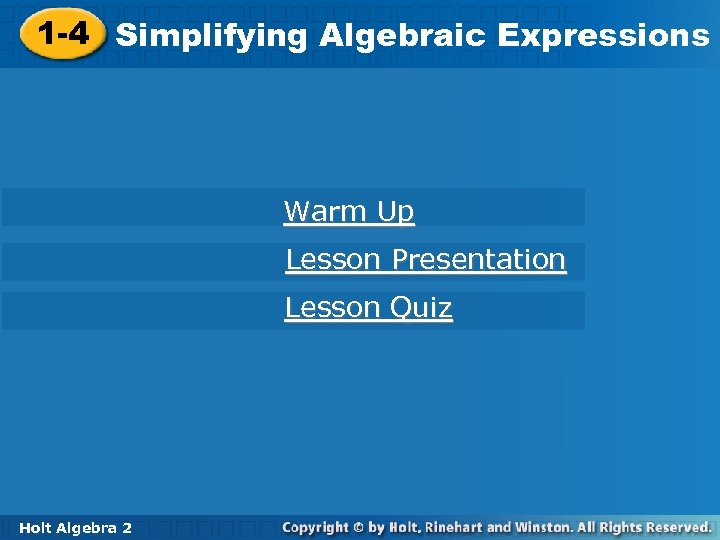1 -4 Simplifying Algebraic Expressions Warm Up Lesson Presentation Lesson Quiz Holt Algebra 2 2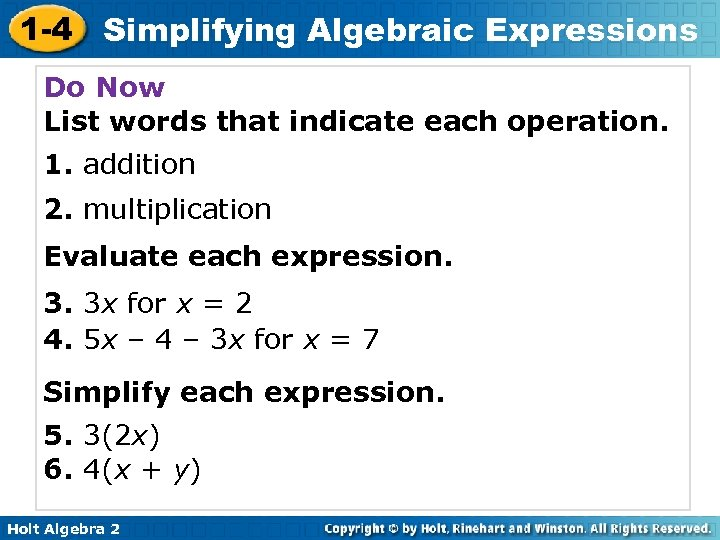1 -4 Simplifying Algebraic Expressions Do Now List words that indicate each operation. 1. addition 2. multiplication Evaluate each expression. 3. 3 x for x = 2 4. 5 x – 4 – 3 x for x = 7 Simplify each expression. 5. 3(2 x) 6. 4(x + y) Holt Algebra 2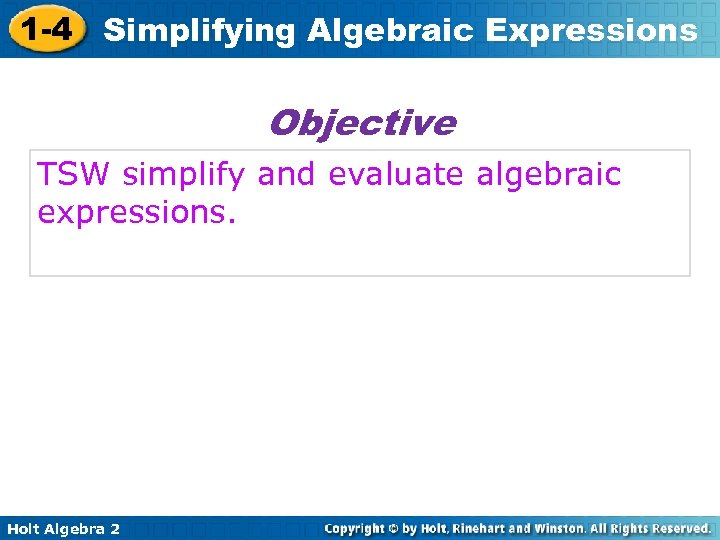1 -4 Simplifying Algebraic Expressions Objective TSW simplify and evaluate algebraic expressions. Holt Algebra 2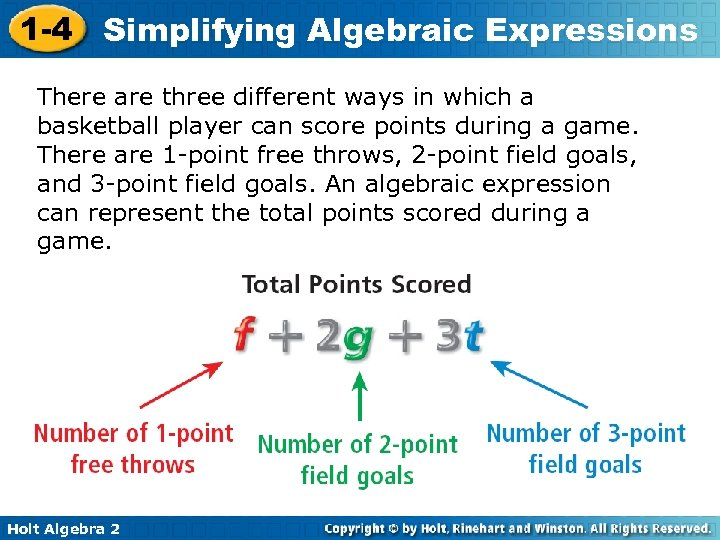1 -4 Simplifying Algebraic Expressions There are three different ways in which a basketball player can score points during a game. There are 1 -point free throws, 2 -point field goals, and 3 -point field goals. An algebraic expression can represent the total points scored during a game. Holt Algebra 2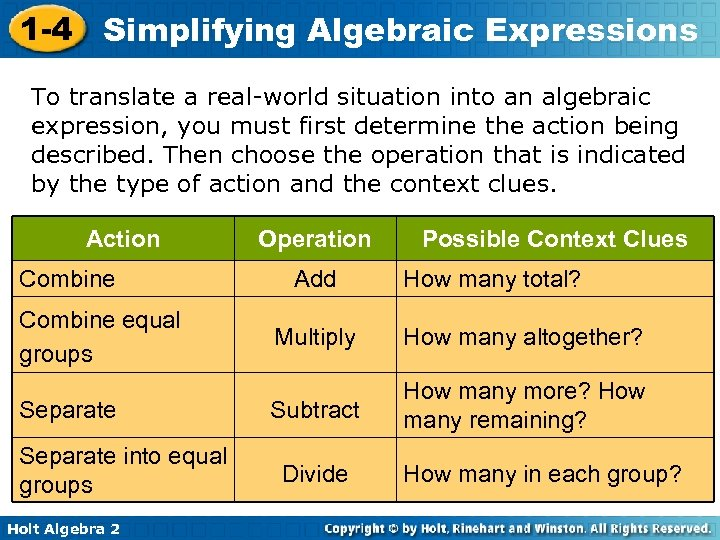1 -4 Simplifying Algebraic Expressions To translate a real-world situation into an algebraic expression, you must first determine the action being described. Then choose the operation that is indicated by the type of action and the context clues. Action Combine equal groups Separate into equal groups Holt Algebra 2 Operation Add Possible Context Clues How many total? Multiply How many altogether? Subtract How many more? How many remaining? Divide How many in each group?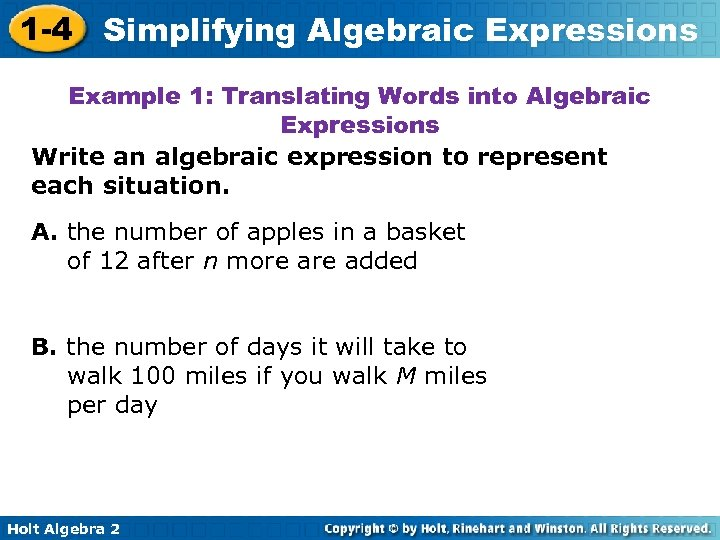1 -4 Simplifying Algebraic Expressions Example 1: Translating Words into Algebraic Expressions Write an algebraic expression to represent each situation. A. the number of apples in a basket of 12 after n more added B. the number of days it will take to walk 100 miles if you walk M miles per day Holt Algebra 2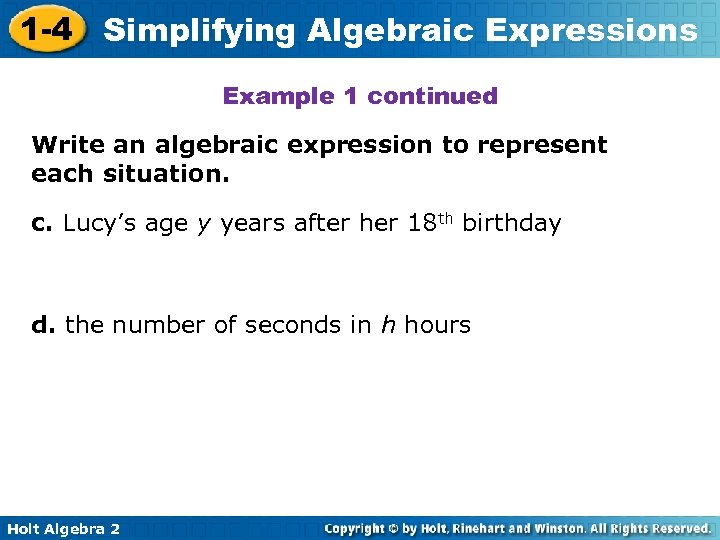1 -4 Simplifying Algebraic Expressions Example 1 continued Write an algebraic expression to represent each situation. c. Lucy’s age y years after her 18 th birthday d. the number of seconds in h hours Holt Algebra 2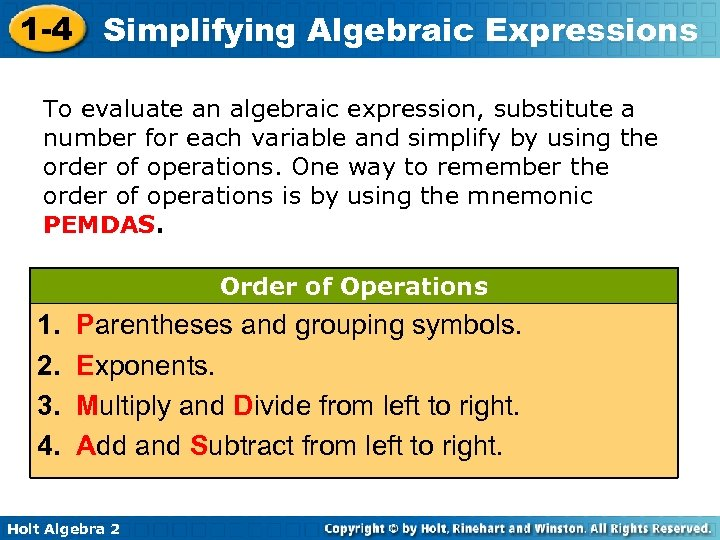1 -4 Simplifying Algebraic Expressions To evaluate an algebraic expression, substitute a number for each variable and simplify by using the order of operations. One way to remember the order of operations is by using the mnemonic PEMDAS. Order of Operations 1. 2. 3. 4. Parentheses and grouping symbols. Exponents. Multiply and Divide from left to right. Add and Subtract from left to right. Holt Algebra 2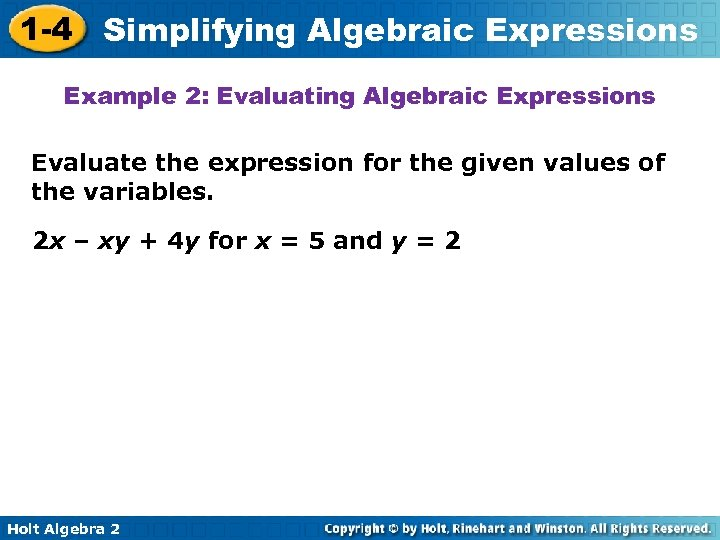1 -4 Simplifying Algebraic Expressions Example 2: Evaluating Algebraic Expressions Evaluate the expression for the given values of the variables. 2 x – xy + 4 y for x = 5 and y = 2 Holt Algebra 2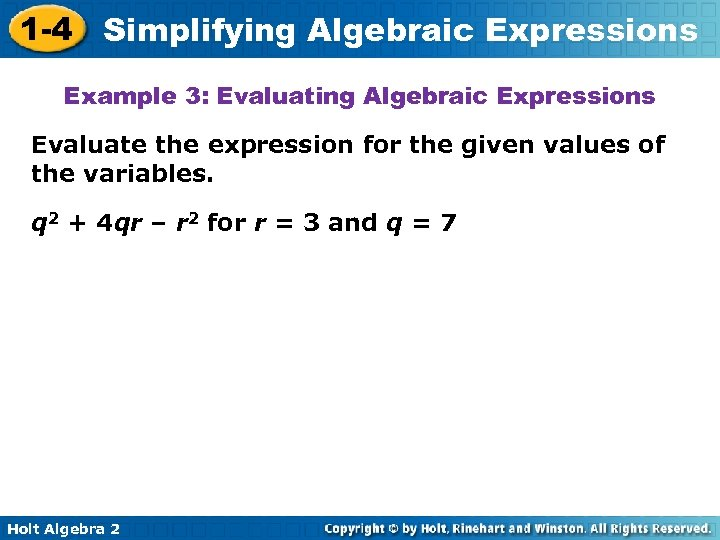1 -4 Simplifying Algebraic Expressions Example 3: Evaluating Algebraic Expressions Evaluate the expression for the given values of the variables. q 2 + 4 qr – r 2 for r = 3 and q = 7 Holt Algebra 2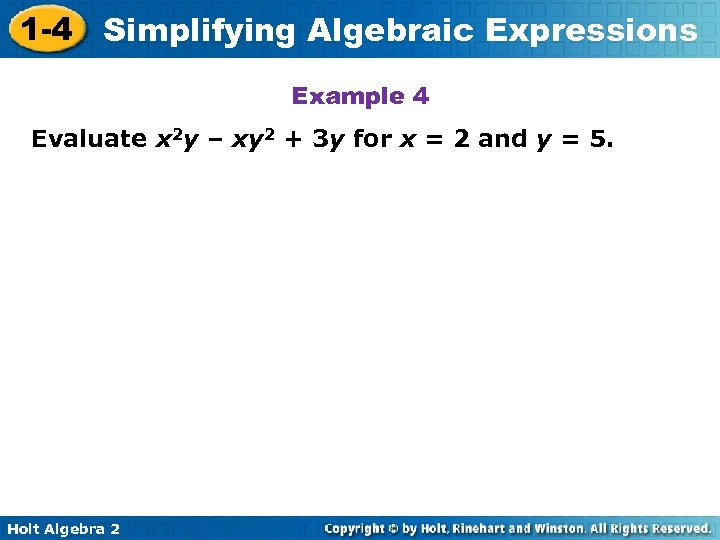1 -4 Simplifying Algebraic Expressions Example 4 Evaluate x 2 y – xy 2 + 3 y for x = 2 and y = 5. Holt Algebra 2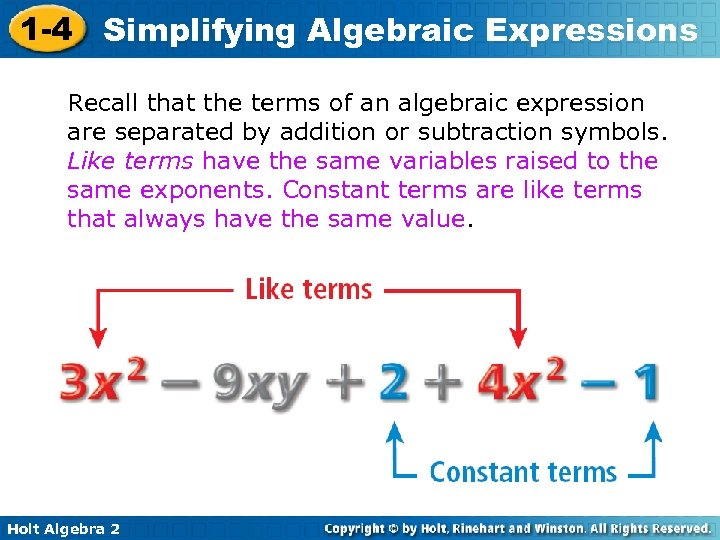1 -4 Simplifying Algebraic Expressions Recall that the terms of an algebraic expression are separated by addition or subtraction symbols. Like terms have the same variables raised to the same exponents. Constant terms are like terms that always have the same value. Holt Algebra 2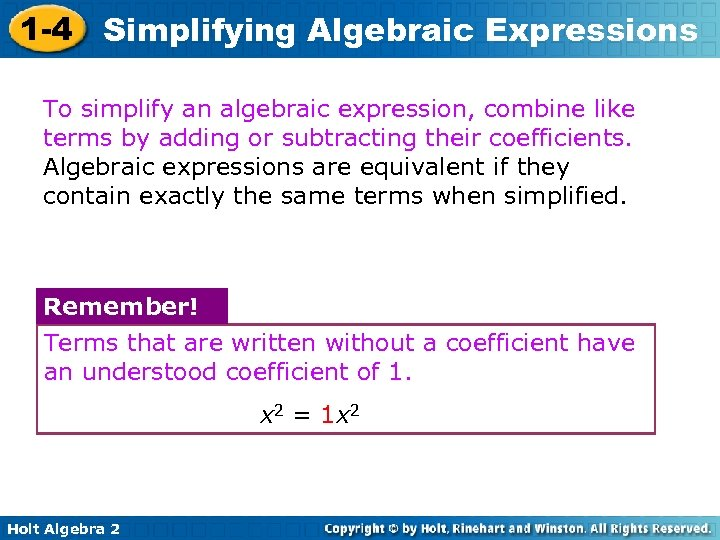1 -4 Simplifying Algebraic Expressions To simplify an algebraic expression, combine like terms by adding or subtracting their coefficients. Algebraic expressions are equivalent if they contain exactly the same terms when simplified. Remember! Terms that are written without a coefficient have an understood coefficient of 1. x 2 = 1 x 2 Holt Algebra 2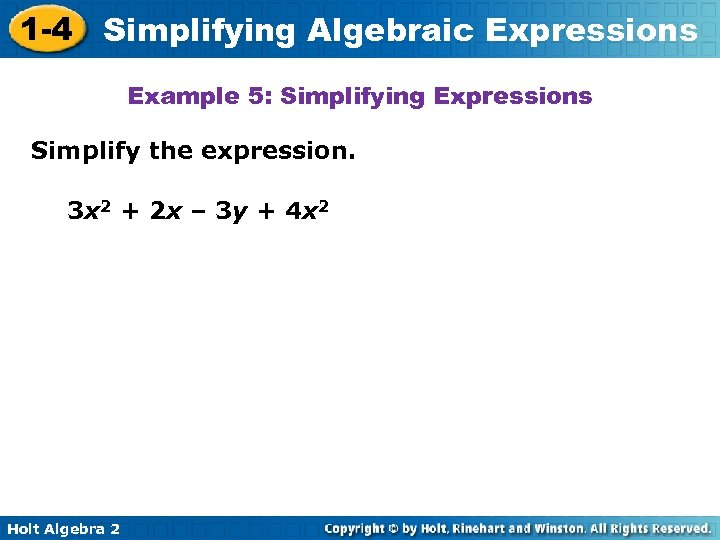1 -4 Simplifying Algebraic Expressions Example 5: Simplifying Expressions Simplify the expression. 3 x 2 + 2 x – 3 y + 4 x 2 Holt Algebra 2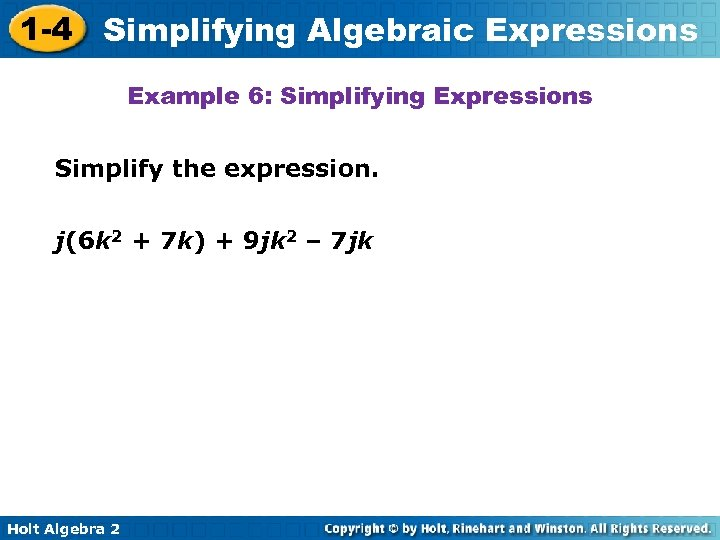1 -4 Simplifying Algebraic Expressions Example 6: Simplifying Expressions Simplify the expression. j(6 k 2 + 7 k) + 9 jk 2 – 7 jk Holt Algebra 2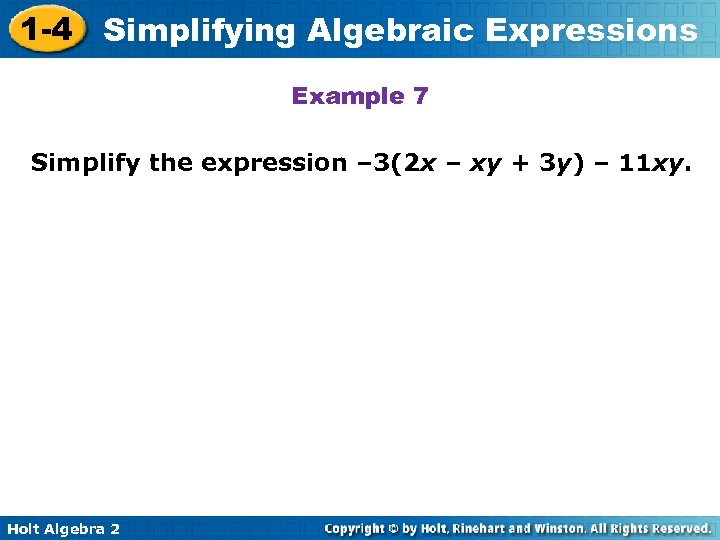1 -4 Simplifying Algebraic Expressions Example 7 Simplify the expression – 3(2 x – xy + 3 y) – 11 xy. Holt Algebra 2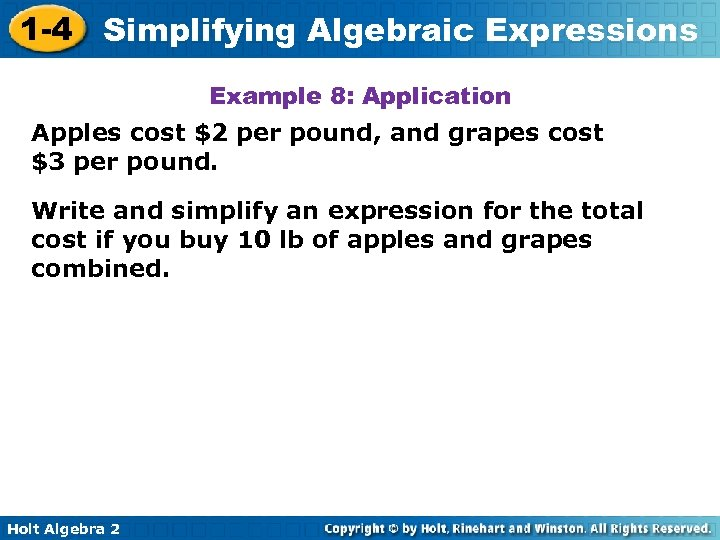1 -4 Simplifying Algebraic Expressions Example 8: Application Apples cost \$2 per pound, and grapes cost \$3 per pound. Write and simplify an expression for the total cost if you buy 10 lb of apples and grapes combined. Holt Algebra 2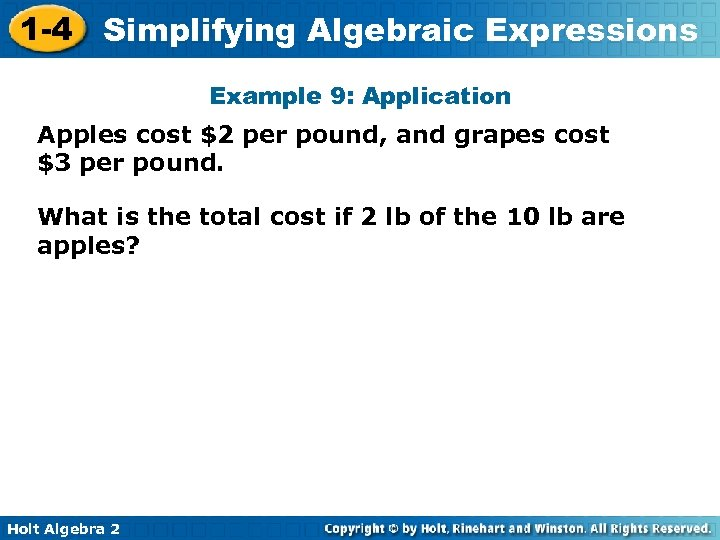1 -4 Simplifying Algebraic Expressions Example 9: Application Apples cost \$2 per pound, and grapes cost \$3 per pound. What is the total cost if 2 lb of the 10 lb are apples? Holt Algebra 2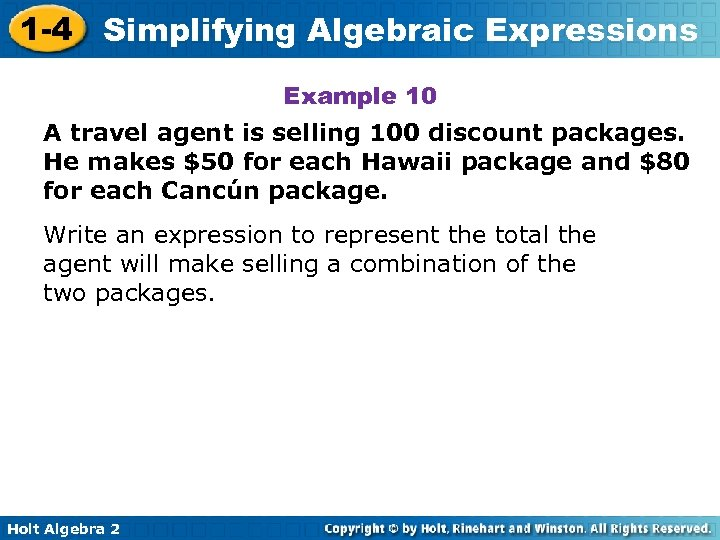1 -4 Simplifying Algebraic Expressions Example 10 A travel agent is selling 100 discount packages. He makes \$50 for each Hawaii package and \$80 for each Cancún package. Write an expression to represent the total the agent will make selling a combination of the two packages. Holt Algebra 2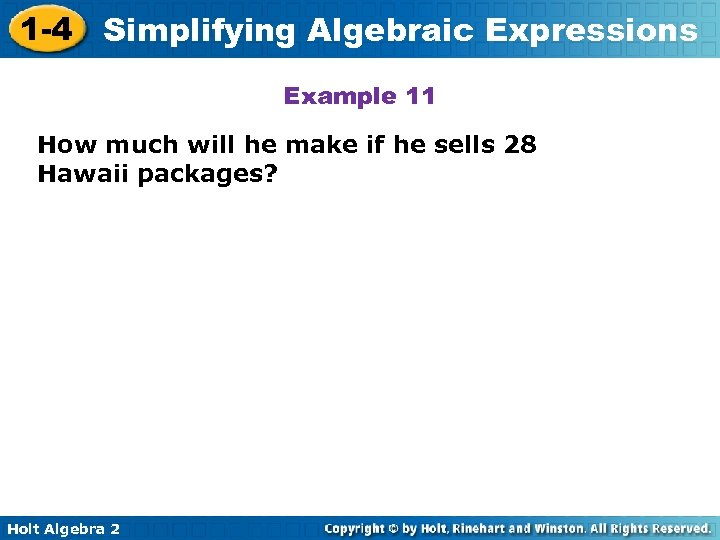1 -4 Simplifying Algebraic Expressions Example 11 How much will he make if he sells 28 Hawaii packages? Holt Algebra 2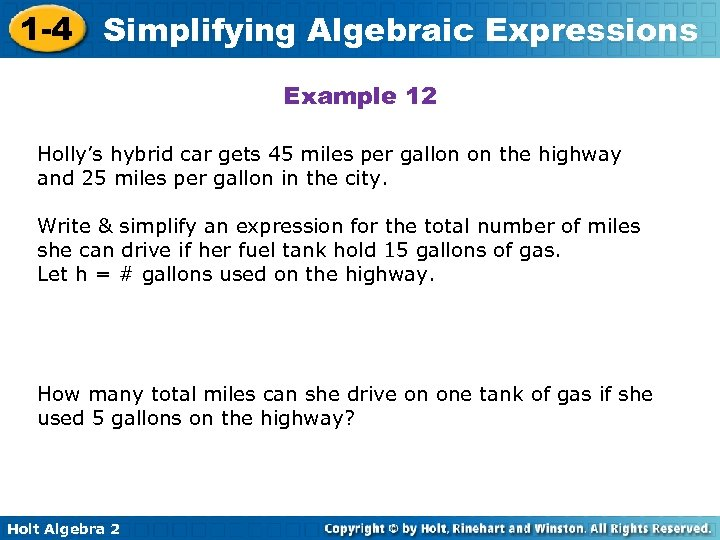1 -4 Simplifying Algebraic Expressions Example 12 Holly’s hybrid car gets 45 miles per gallon on the highway and 25 miles per gallon in the city. Write & simplify an expression for the total number of miles she can drive if her fuel tank hold 15 gallons of gas. Let h = # gallons used on the highway. How many total miles can she drive on one tank of gas if she used 5 gallons on the highway? Holt Algebra 2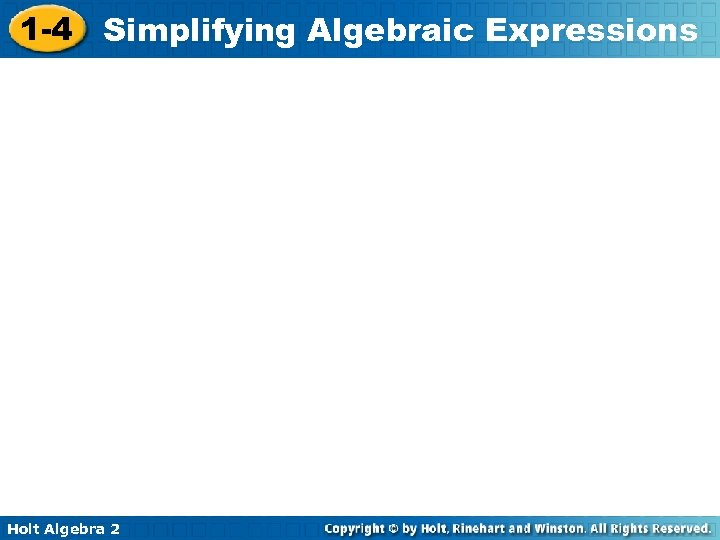1 -4 Simplifying Algebraic Expressions Holt Algebra 2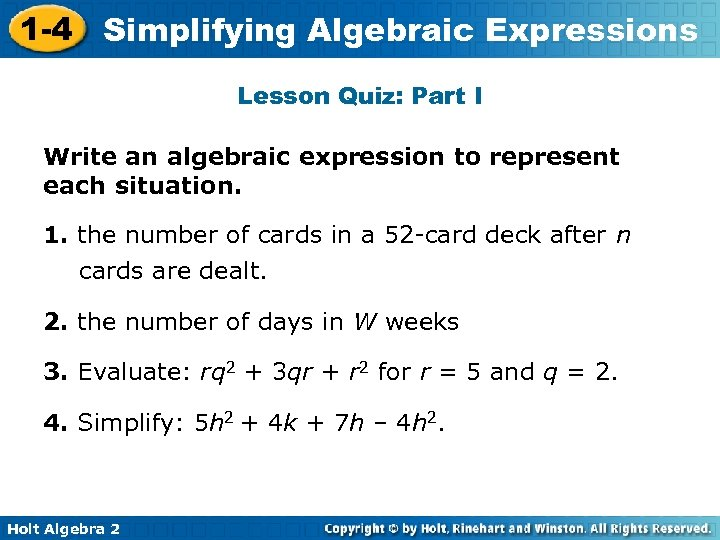1 -4 Simplifying Algebraic Expressions Lesson Quiz: Part I Write an algebraic expression to represent each situation. 1. the number of cards in a 52 -card deck after n cards are dealt. 2. the number of days in W weeks 3. Evaluate: rq 2 + 3 qr + r 2 for r = 5 and q = 2. 4. Simplify: 5 h 2 + 4 k + 7 h – 4 h 2. Holt Algebra 2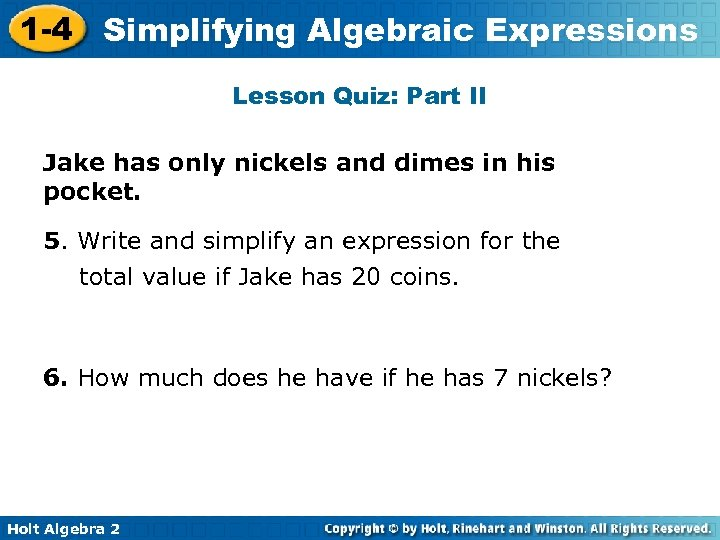1 -4 Simplifying Algebraic Expressions Lesson Quiz: Part II Jake has only nickels and dimes in his pocket. 5. Write and simplify an expression for the total value if Jake has 20 coins. 6. How much does he have if he has 7 nickels? Holt Algebra 2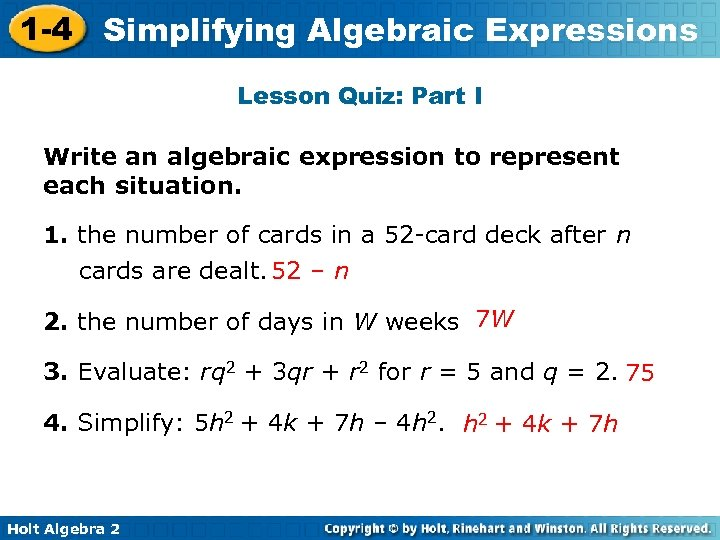1 -4 Simplifying Algebraic Expressions Lesson Quiz: Part I Write an algebraic expression to represent each situation. 1. the number of cards in a 52 -card deck after n cards are dealt. 52 – n 2. the number of days in W weeks 7 W 3. Evaluate: rq 2 + 3 qr + r 2 for r = 5 and q = 2. 75 4. Simplify: 5 h 2 + 4 k + 7 h – 4 h 2. h 2 + 4 k + 7 h Holt Algebra 2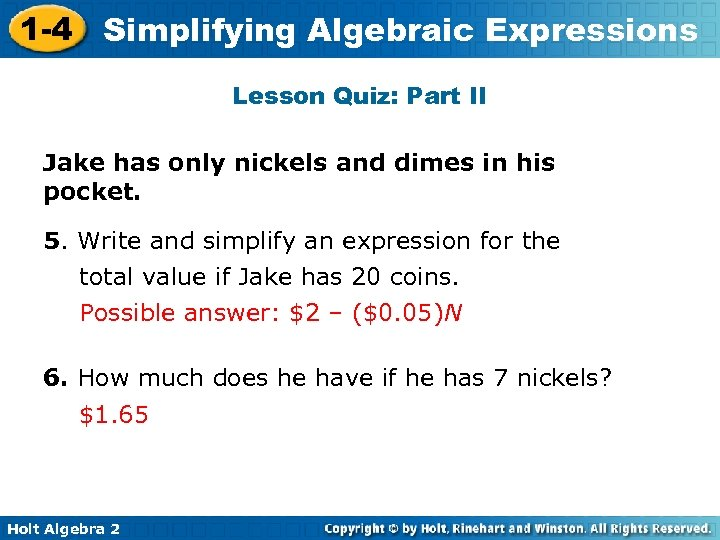1 -4 Simplifying Algebraic Expressions Lesson Quiz: Part II Jake has only nickels and dimes in his pocket. 5. Write and simplify an expression for the total value if Jake has 20 coins. Possible answer: \$2 – (\$0. 05)N 6. How much does he have if he has 7 nickels? \$1. 65 Holt Algebra 2